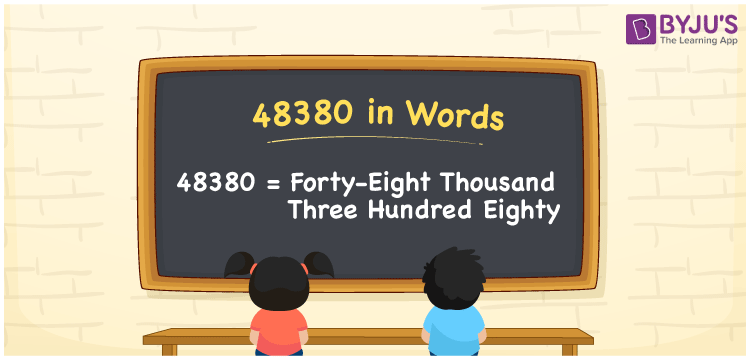# 48380 in Words

48380 in words is Forty-eight Thousand Three Hundred Eighty. For example, if you spend Rs. 48380 in a month, you can write, “I have spent Rs. Forty-eight Thousand Three Hundred Eighty in a month”. In general, the number name of any number can be written using the ones, tens, hundreds, and thousands place of a number. Thus, the place value chart is useful for writing the number 48380 in words.

 48380 in words Forty-eight Thousand Three Hundred Eighty Forty-eight Thousand Three Hundred Eighty in Numbers 48380

## 48380 in English Words## How to Write 48380 in Words?

We can convert 48380 to words using a place value chart. This can be done as follows. The number 48380 has 5 digits, so let’s make a chart that shows the place value up to 5 digits.

 Ten thousands Thousands Hundreds Tens Ones 4 8 3 8 0

Thus, we can write the expanded form as:

4 × Ten thousand + 8 × Thousand + 3 × Hundred + 8 × Ten + 0 × One

= 4 × 10000 + 8 × 1000 + 3 × 100 + 8 × 10 + 0 × 1

= 48380

= Forty-eight Thousand Three Hundred Eighty

48380 is the natural number that is succeeded by 48379 and preceded by 48381.

48380 in words – Forty-eight Thousand Three Hundred Eighty

Is 48380 an odd number? – No.

Is 48380 an even number? – Yes

Is 48380 a perfect square number? – No

Is 48380 a perfect cube number? – No

Is 48380 a prime number? – No

Is 48380 a composite number? – Yes

## Frequently Asked Questions on 48380 in Words

Q1

### How to write 48380 in words?

48380 in English is written as “Forty-eight Thousand Three Hundred Eighty”.
Q2

### Is the number 48380 an odd number?

No, the number 48380 is not an odd number.
Q3

### Is 48380 a composite number?

Yes, 48380 is a composite number.